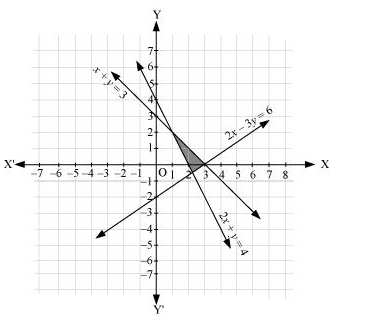# Solve the following system of inequalities graphically: 2x + y≥ 4, x + y ≤ 3, 2x – 3y ≤ 6

Question:

Solve the following system of inequalities graphically: 2x + y 4, x + y  3, 2x – 3y  6

Solution:

$2 x+y \geq 4 \ldots(1)$

$x+y \leq 3 \ldots(2)$

$2 x-3 y \leq 6$  $\ldots(3)$

The graph of the lines, 2x + y= 4, x + y = 3, and 2x – 3y = 6, are drawn in the figure below.

Inequality (1) represents the region above the line, 2x + y= 4 (including the line 2x + y= 4). Inequality (2) represents the region below the line,

x + y = 3 (including the line x + y = 3). Inequality (3) represents the region above the line, 2x – 3y = 6 (including the line 2x – 3y = 6).

Hence, the solution of the given system of linear inequalities is represented by the common shaded region including the points on the respective lines as follows.